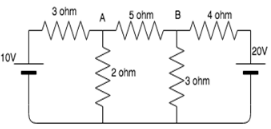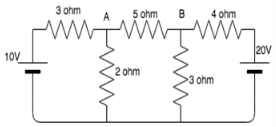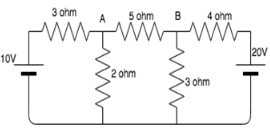# Test: Maximum Power Transfer

## 10 Questions MCQ Test Network Theory (Electric Circuits) | Test: Maximum Power Transfer

Description
Attempt Test: Maximum Power Transfer | 10 questions in 10 minutes | Mock test for Electrical Engineering (EE) preparation | Free important questions MCQ to study Network Theory (Electric Circuits) for Electrical Engineering (EE) Exam | Download free PDF with solutions
QUESTION: 1

### The maximum power drawn from source depends on__________

Solution:

The maximum power transferred is equal to:
I2RL, when load resistance is variable.
Es2/4RL, when load impedance is variable.
In both the cases, the maximum power depends on the load resistance.

QUESTION: 2

### The maximum power is delivered to a circuit when source resistance is __________ load resistance.

Solution:

The circuit can draw maximum power only when source resistance is equal to the load resistance.

QUESTION: 3

### If source impedance is a complex number Z, then load impedance is equal to _________

Solution:

When Source impedance is equal to Z, its load impedance is the complex conjugate of Z which is Z’. Only under this condition maximum power can be drawn from the circuit.

QUESTION: 4

If ZL=Zs’, then RL=?

Solution:

Rs is the real part of the complex number ZL. Hence when we find the complex conjugate the real part remains the same whereas the complex part acquires a negative sign.

QUESTION: 5

Calculate the value of RL across A and B.Solution:

On shorting the voltage sources:
RL=3||2+4||5.

QUESTION: 6

Calculate Vba.​Solution:

The two nodal equations are:
(VA-10)/3+VA/2=0
(VB-20)/4+VB/3=0
On solving the two equations, we get VA=4V, VB=8.571V.
Vba=VA-VB= 4.57V.

QUESTION: 7

Calculate the maximum power transferred.Solution:

Explanation : On shorting the voltage sources:

RL = 3||2+4||3

= 1.20 + 1.71

= 2.91 ohm.

The two nodal equations are:

(VA-10)/3 + VA/2 = 0

(VB-20)/4 + VB/3 = 0

On solving the two equations, we get VA=4V, VB=8.571V.

VAB = VA - VB

= 4V – 8.571V

= -4.57V.

Eth = 4.57V

The maximum power transferred = Eth2/4RL. Substituting the given values in the formula, we get Pmax = 1.79W

QUESTION: 8

Does maximum power transfer imply maximum efficiency?

Solution:

Maximum power transfer does not imply maximum efficiency. If the load resistance is smaller than source resistance, power dissipated at the load is reduced while most of the power is dissipated at the source then the efficiency becomes lower.

QUESTION: 9

Under the condition of maximum power efficiency is?

Solution:

Efficiency=output/input*100.
Under maximum power conditions:
Output=I2RL; Input=2*I2RL.
Thus efficiency=50%.

QUESTION: 10

Name some devices where maximum power has to be transferred to the load rather than maximum efficiency.

Solution:

Maximum power transfer to the load is preferred over maximum efficiency in both amplifiers and communication circuits since in both these cases the output voltage is more than the input.Use Code STAYHOME200 and get INR 200 additional OFF Use Coupon Code